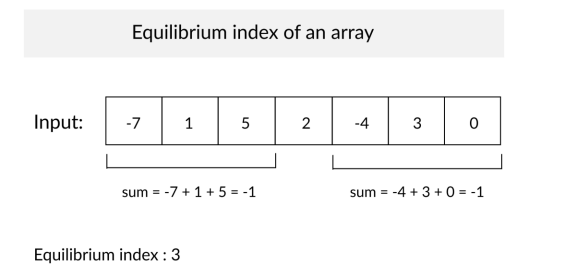×

Search anything:

# Equilibrium Index: Find index such that sum of left sub-array = right sub-array

#### List of Mathematical Algorithms Algorithms prefix sum array Interview Problems on ArrayGet this book -> Problems on Array: For Interviews and Competitive Programming

For a given array, we need to find an index such that sum of left sub-array = right sub-array also called the Equilibrium Index.
Equilibrium index is the index of the element in the array such that the sum of all the elements left to the index is equal to the sum of all the elements right to the index .

(A + A + … + A[i-1]) = (A[i+1] + A[i+2] + … + A[n-1]), where 0 < i < n-1input:
[3, 4, 1, 5, 2, 6]
output:
3
Explanation:
The left and right sub array for index '3' is [3, 4, 1] and [2, 6] respectively and the sum of both the subarray is 8.
In the above example '3' is the equillibrium index = 4.

We have explored two techniques:

• Brute force method O(N^2) time
• Using Prefix and Suffix array O(N) time

# Brute Force Method

Algorithm:

The steps in the Brute force algorithm are:

Step 1: Traverse each index in the array
Step 2: For each index check if the sum of the elements left to it is equal to the sum of elements right to it.
Step 3: If the sum of left and right sub array for the current index is equal then print it and go to step 5.
Step 4: If sum of left and right sub array for the current index is not equal move to the next element and goto step 3.
Step 5: Exit

Implementation:

The implementation of the brute force approach in C++:

``````// Part of iq.OpenGenus.org
#include<iostream>
using namespace std;
//fun to calculate the subarray sum
int sumSubArray(int arr[],int start,int end){
int sum = 0;
for(int i=start;i<=end;i++)
sum += arr[i];
return sum;
}
//driver code
int main(){

int arr[] = { 1,6,2,7 };
int n = sizeof(arr) / sizeof(arr);

//brute force algorithm
//we check for every index in array
for(int i=1;i<n-1;i++){

//calculate the left and right subarray sum
int left_sum=sumSubArray(a,0,i-1);
int right_sum=sumSubArray(a,i+1,n-1);

//check if curr index if eqillibrium index
if(left_sum==right_sum){
cout<<i; // eqillibrium index
break;
}

}
return 0;
}
``````

Complexity Analysis:
In this algorithm it takes O(n) time to traverse every index and O(n) time to calculate the subarray sum for each index so the total time complexity is O(n^2) and as we do not take any extra space ,the space complexity is constant.

time complexity: O(n^2)
space complexity: O(1)

This is not an efficient solution so let's see another algorithm for this problem.

# Using Prefix and Suffix Arrays

Algorithm:

Step 1: Traverse the array from left to right and calculate and store the cumulative sum at every element in an array. Let this array be prefix sum array.
Step 2: The last element in this prefix array is the sum of all the elements of the array.
Step 3: Take a new array and let this sum be its first element. At each element, subtract the element value from the previously calculated value and store it in the array.Let this array be suffix sum array.
Step 4: Compare the two arrays and find the index at which both the arrays have identical elements and print this index.

Given array: 1 6 2 7

Prefix Sum array: 1 7 9 16
Suffix Sum array: 16 15 9 7

Explanation:
we traverse both arrays.
at index 2 they both have identical element (9).
therefore 2 is the equillibrium index.

Implementation:

The implementation of the efficient approach in C++:

``````// Part of iq.OpenGenus.org
#include <iostream>
using namespace std;

int equillibrium_index(int arr[], int n)
{
// making prefix sum array
int prefixSum[n];
prefixSum = arr;
for (int i = 1; i < n; i++)
prefixSum[i] = prefixSum[i - 1] + arr[i];

// making suffix sum array
int suffixSum[n];
suffixSum = prefixSum[n-1];
for(int i=1;i<n;i++){
suffixSum[i] = suffixSum[i-1] - arr[i-1];
}

// finding index at which both prefix and suffix sum array have same element
for (int i = 1; i < n - 1; i++)
if (prefixSum[i] == suffixSum[i])
return arr[i];//return the equillibrium index

//if there is no euillibrium index
return -1;
}

// Driver code
int main()
{
int arr[] = { 1,6,2,7 };
int n = sizeof(arr) / sizeof(arr);
cout << equillibrium_index(arr, n);
return 0;
}
``````

Complexity Analysis:

In the above algorithm, it takes O(n) time to form prefix sum array,O(n) time to form suffix sum array and O(n) time to find the index with identical elements in the two arrays. thus, the total time complexity is O(n).
As we have created two more arrays in this approach each of size n, the total space complexity is O(n).

time complexity: O(n)
space complexity: O(n)

Optimization: Avoid using Suffix Array

In this approach, we can also avoid creating the suffix array as the values can be generated by subtracting prefix sum from total sum.

``````suffix[i] = prefix[N] - prefix[i]
``````

This is because:

``````prefix[N] = a1 + a2 + ... + aN

prefix[i] = a1 + a2 + ... + ai

Therefore, prefix[N] - prefix[i] =
a(i+1) + ... + aN

Hence, suffix[i] = prefix[N] - prefix[i]
``````

It is clear that this approach is better (as it takes O(N) time) that the brute force approach which takes O(n^2) time.

## Question

#### 3 5 4 2 3 is the equillibrium index because at index 3 the sum of left and right subarray is 16.#### Shreya Shah

Intern at OpenGenus, B. Tech Student at Jabalpur Engineering College (JEC) in Information Technology, 2023.

Equilibrium Index: Find index such that sum of left sub-array = right sub-array